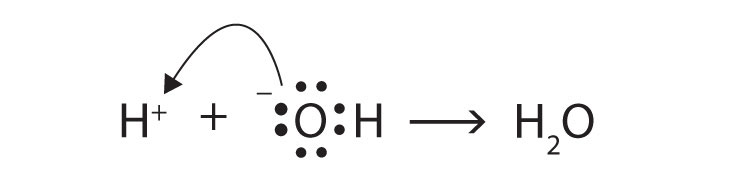## 10.8 End-of-Chapter Material

### Chapter Summary

To ensure that you understand the material in this chapter, you should review the meanings of the bold terms in the following summary and ask yourself how they relate to the topics in the chapter.

The earliest chemical definition of an acid, the Arrhenius definition, says that an acid is a compound that increases the amount of hydrogen ion (H+) in aqueous solution. An Arrhenius base is a compound that increases the amount of hydroxide ion (OH) in aqueous solution. While most bases are named as ionic hydroxide compounds, aqueous acids have a naming system unique to acids. Acids and bases react together in a characteristic chemical reaction called neutralization, in which the products are water and a salt. The principles of stoichiometry, along with the balanced chemical equation for a reaction between an acid and a base, can be used to determine how much of one compound will react with a given amount of the other.

A Brønsted-Lowry acid is any substance that donates a proton to another substance. A Brønsted-Lowry base is any substance that accepts a proton from another substance. The reaction of ammonia with water to make ammonium ions and hydroxide ions can be used to illustrate Brønsted-Lowry acid and base behavior.

Some compounds can either donate or accept protons, depending on the circumstances. Such compounds are called amphiprotic. Water is one example of an amphiprotic compound. One result of water being amphiprotic is that a water molecule can donate a proton to another water molecule to make a hydronium ion and a hydroxide ion. This process is called the autoionization of water and occurs in any sample of water.

Not all acids and bases are equal in chemical strength. A strong acid is an acid whose molecules are all dissociated into ions in aqueous solution. Hydrochloric acid is an example of a strong acid. Similarly, a strong base is a base whose molecules are dissociated into ions in aqueous solution. Sodium hydroxide is an example of a strong base. Any acid or base whose molecules are not all dissociated into ions in aqueous solution is a weak acid or a weak base. Solutions of weak acids and weak bases reach a chemical equilibrium between the un-ionized form of the compound and the dissociated ions. It is a dynamic equilibrium because acid and base molecules are constantly dissociating into ions and reassociating into neutral molecules.

The pH scale is a scale used to express the concentration of hydrogen ions in solution. A neutral solution, neither acidic nor basic, has a pH of 7. Acidic solutions have a pH lower than 7, while basic solutions have a pH higher than 7.

Buffers are solutions that resist dramatic changes in pH when an acid or a base is added to them. They contain a weak acid and a salt of that weak acid, or a weak base and a salt of that weak base. When a buffer is present, any strong acid reacts with the anion of the salt, forming a weak acid and minimizing the presence of hydrogen ions in solution. Any strong base reacts with the weak acid, minimizing the amount of additional hydroxide ions in solution. However, buffers only have limited capacity; there is a limit to the amount of strong acid or strong base any given amount of buffer will react with.

1. The properties of a 1.0 M HCl solution and a 1.0 M HC2H3O2 solution are compared. Measurements show that the hydrochloric acid solution has a higher osmotic pressure than the acetic acid solution. Explain why.

2. Of a 0.50 M HNO3 solution and a 0.50 M HC2H3O2 solution, which should have the higher boiling point? Explain why.

3. The reaction of sulfuric acid [H2SO4(aq)] with sodium hydroxide [NaOH(aq)] can be represented by two separate steps, with only one hydrogen ion reacting in each step. Write the chemical equation for each step.

4. The reaction of aluminum hydroxide [Al(OH)3(aq)] with hydrochloric acid [HCl(aq)] can be represented by three separate steps, with only one hydroxide ion reacting in each step. Write the chemical equation for each step.

5. A friend brings you a small sample of an unknown chemical. Assuming that the chemical is soluble in water, how would you determine if the chemical is an acid or a base?

6. A neutral solution has a hydrogen ion concentration of about 1 × 10−7 M. What is the concentration of the hydroxide ion in a neutral solution?

7. The Lewis definitions of an acid and a base are based on electron pairs, not protons. A Lewis acid is an electron pair acceptor, while a Lewis base is an electron pair donor. Use Lewis diagrams to show that

H+(aq) + OH(aq) → H2O(ℓ)

is an acid-base reaction in the Lewis sense as well as in the Arrhenius and Brønsted-Lowry senses.

8. Given the chemical reaction

NH3(g) + BF3(g) → NH3—BF3(s)

show that the reaction illustrated by this equation is an acid-base reaction if we use the Lewis definitions of an acid and a base (see Exercise 7). The product contains a bond between the N and B atoms.

4.